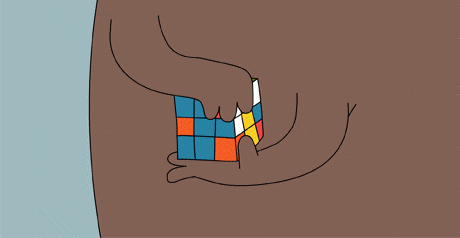# Happy 40th anniversary Rubik's Cube!Today the Rubik's cube celebrates $40$ years of bringing joy to children and adults alike.Besides the pleasure of solving it,it also has a lot of mathematics to it too.

Consider a solved $3 \times 3$ Rubik's cube. The six faces of the cube are named $FRONT$, $BACK$, $UP$, $DOWN$, $LEFT$ and $RIGHT$ respectively. An elementary move of the Rubik's cube is rotating a face by $90°$ clockwise or $90°$ anticlockwise. Any valid state of the Rubik's cube can be reached by applying these elementary operations one after the other.

An elementary move is denoted in the following fashion. If a given face is rotated by $90°$ clockwise about the axis passing from the center of the face to the center of the cube, the move is denoted by the first letter of the name of the face. If the rotation is anticlockwise by $90°$, the letter is followed by an apostrophe (').These are all the elementary moves: $R R' U U' L L' D D' B B' F F'$

Given a single string of elementary moves $S$ let $F(s)$ denote the number of times the sequence should be applied repeatedly to the solved cube to get back the original cube.

Consider the following ten strings $D F'$ $B R' U' F' D'$ $B' F' B'$ $F L' U B$ $F B U L'$ $D U F$ $F F'$ $U' B' L$ $B' R' R' U' F'$ $B L' B L'$

Find $\sum F(S)$ for the ten strings above.

Explicit examples

$F(LB')=63$

$F(RR')=1$

$F(FUB)=90$

×